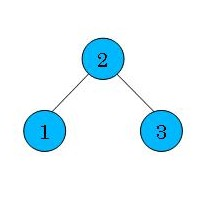```Simple example of Graph, consisting of three vertices and two edges
('test.scm' in the package). Vertices are labelled as 1, 2, and 3, and edges
as (1, 2) and (2, 3). In this Graph, Hamilton path is defined as (1, 2, 3)
or (3, 2, 1).
```